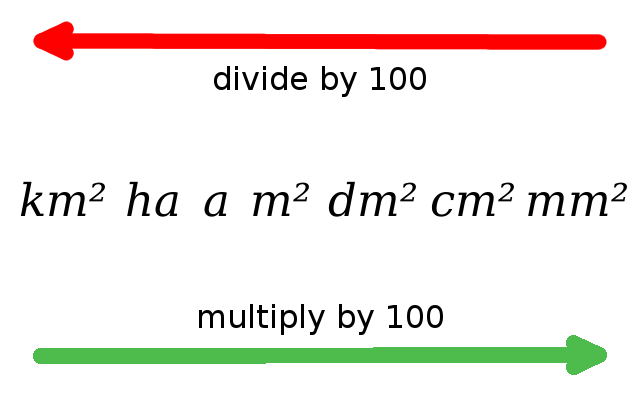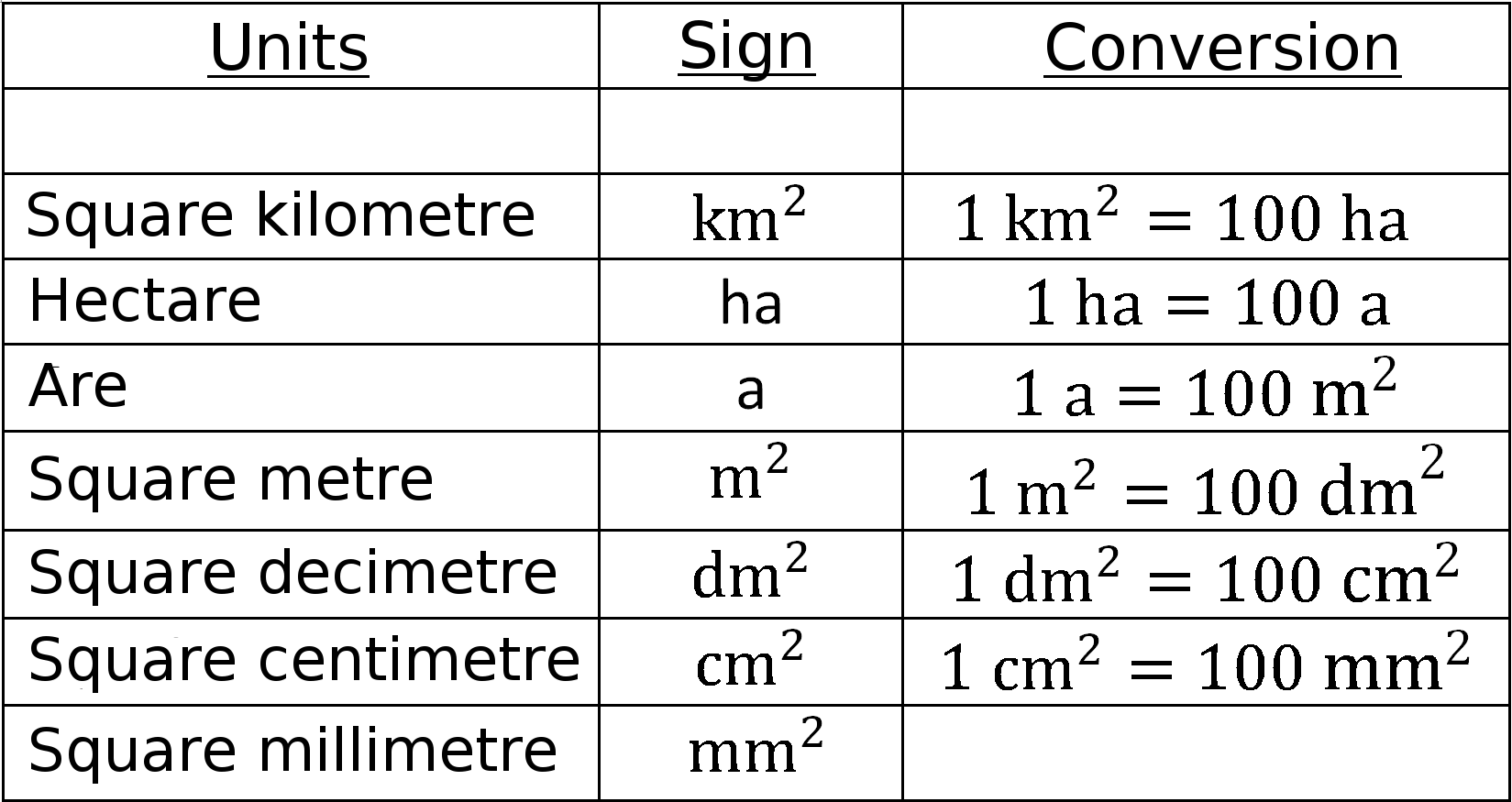Math Geometric formulas Definition area

# Area

The area (formula symbol: $A$) is the size of a two-dimensional geometric figure.
The unit is expressed in square (e.g. $m^{ 2 }$).

Three-dimensional figures also have a so called surface area, which denotes the size of the entire surface.
The unit is also indicated in square here.

i

### Hint

Each two-dimensional figure has its own formula for calculating the area. Formulas see formulary 2D.

For formulas on the surface area of objects, see formulas 3D.

### Conversion